# Angles and Their Measure Angles An angle is

• Slides: 18Angles and Their MeasureAngles An angle is formed by two rays that have a common endpoint called the vertex. One ray is called the initial side and the other the terminal side. The arrow near the vertex shows the direction and the amount of rotation from the initial side to the terminal side. C A è Initial Side Terminal Side B VertexAngles of the Rectangular Coordinate System An angle is in standard position if • its vertex is at the origin of a rectangular coordinate system and • its initial side lies along the positive x-axis. y y is positive Terminal Side Vertex Initial Side x Terminal Side is negative Positive angles rotate counterclockwise. Negative angles rotate clockwise.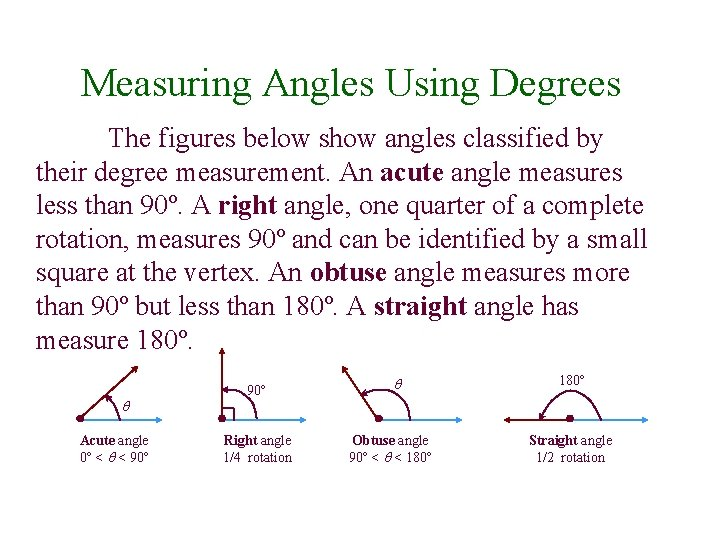Measuring Angles Using Degrees The figures below show angles classified by their degree measurement. An acute angle measures less than 90º. A right angle, one quarter of a complete rotation, measures 90º and can be identified by a small square at the vertex. An obtuse angle measures more than 90º but less than 180º. A straight angle has measure 180º. Acute angle 0º < < 90º Right angle 1/4 rotation Obtuse angle 90º < < 180º Straight angle 1/2 rotation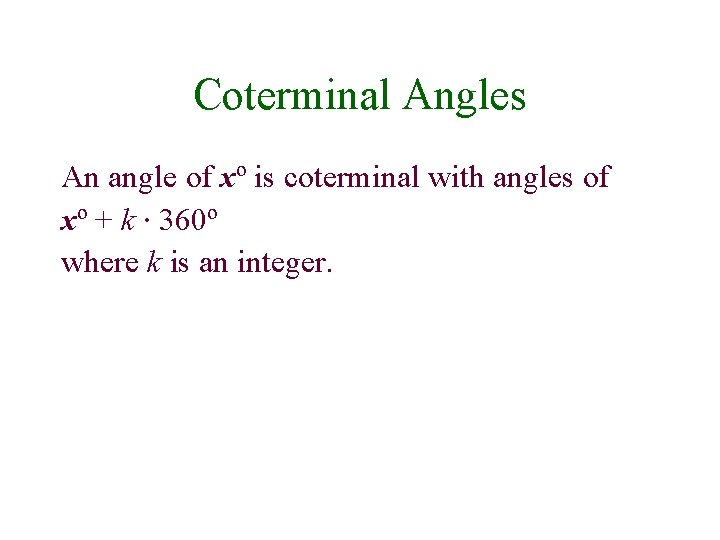Coterminal Angles An angle of xº is coterminal with angles of xº + k · 360º where k is an integer.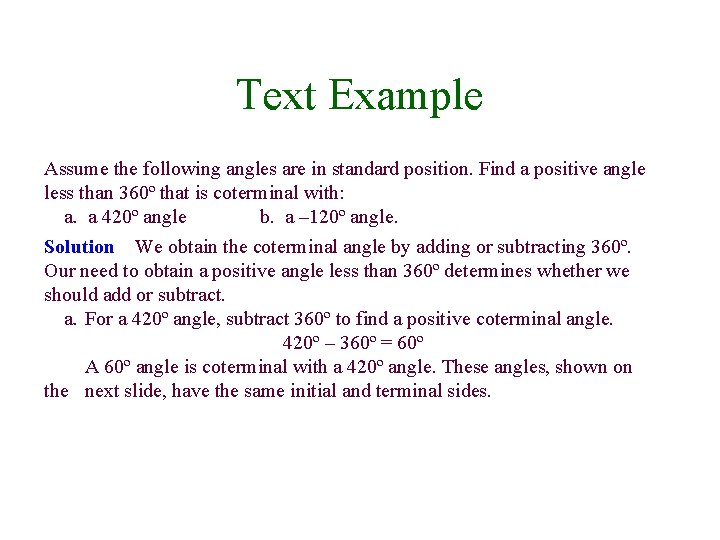Text Example Assume the following angles are in standard position. Find a positive angle less than 360º that is coterminal with: a. a 420º angle b. a – 120º angle. Solution We obtain the coterminal angle by adding or subtracting 360º. Our need to obtain a positive angle less than 360º determines whether we should add or subtract. a. For a 420º angle, subtract 360º to find a positive coterminal angle. 420º – 360º = 60º A 60º angle is coterminal with a 420º angle. These angles, shown on the next slide, have the same initial and terminal sides.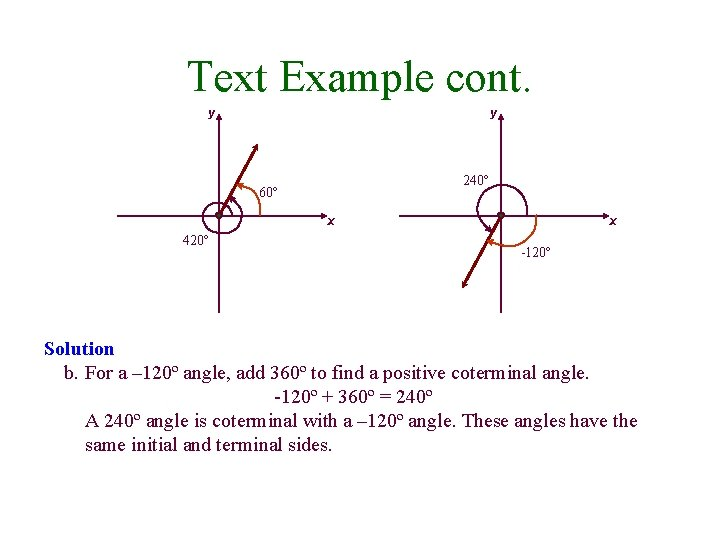Text Example cont. y y 240º 60º x 420º x -120º Solution b. For a – 120º angle, add 360º to find a positive coterminal angle. -120º + 360º = 240º A 240º angle is coterminal with a – 120º angle. These angles have the same initial and terminal sides.Finding Complements and Supplements • For an xº angle, the complement is a 90º – xº angle. Thus, the complement’s measure is found by subtracting the angle’s measure from 90º. • For an xº angle, the supplement is a 180º – xº angle. Thus, the supplement’s measure is found by subtracting the angle’s measure from 180º.Definition of a Radian • One radian is the measure of the central angle of a circle that intercepts an arc equal in length to the radius of the circle.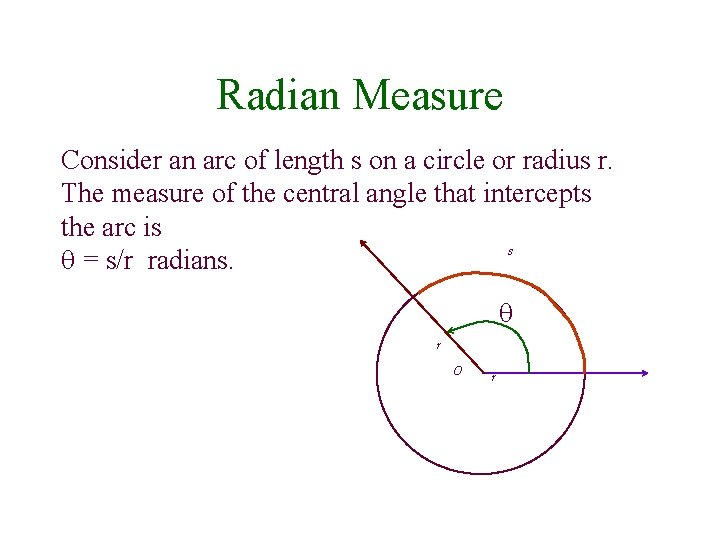Radian Measure Consider an arc of length s on a circle or radius r. The measure of the central angle that intercepts the arc is s = s/r radians. r O r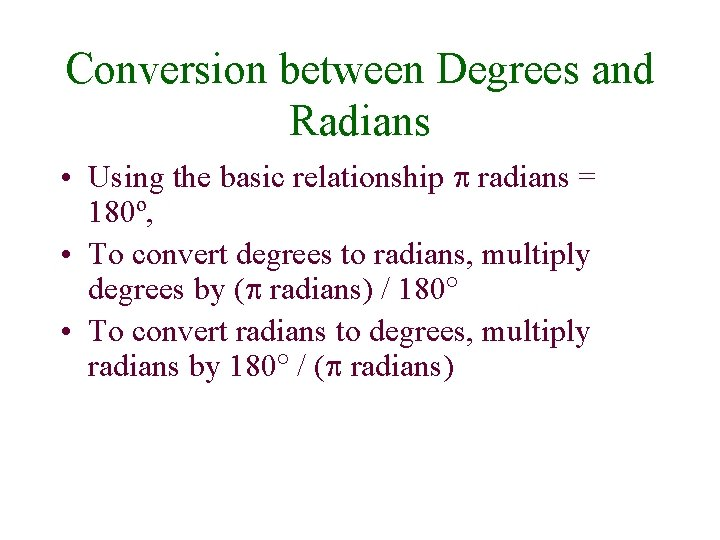Conversion between Degrees and Radians • Using the basic relationship radians = 180º, • To convert degrees to radians, multiply degrees by ( radians) / 180 • To convert radians to degrees, multiply radians by 180 / ( radians)Example Convert each angle in degrees to radians 40º 75º -160ºExample cont. Solution: • 40º = 40* /180 = 2 /9 • 75º = 75* /180 = 5 /12 • -160º = -160* /180 = -8 /9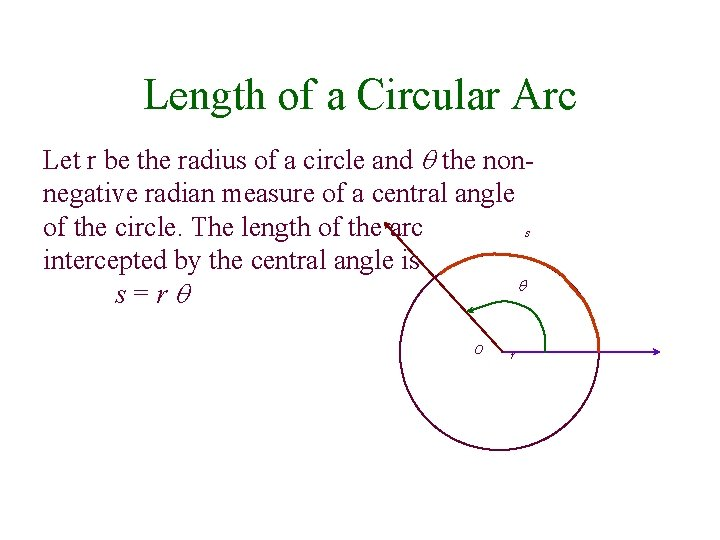Length of a Circular Arc Let r be the radius of a circle and the nonnegative radian measure of a central angle of the circle. The length of the arc s intercepted by the central angle is s=r O rExample A circle has a radius of 7 inches. Find the length of the arc intercepted by a central angle of 2 /3 Solution: s = (7 inches)*(2 /3) =14 /3 inches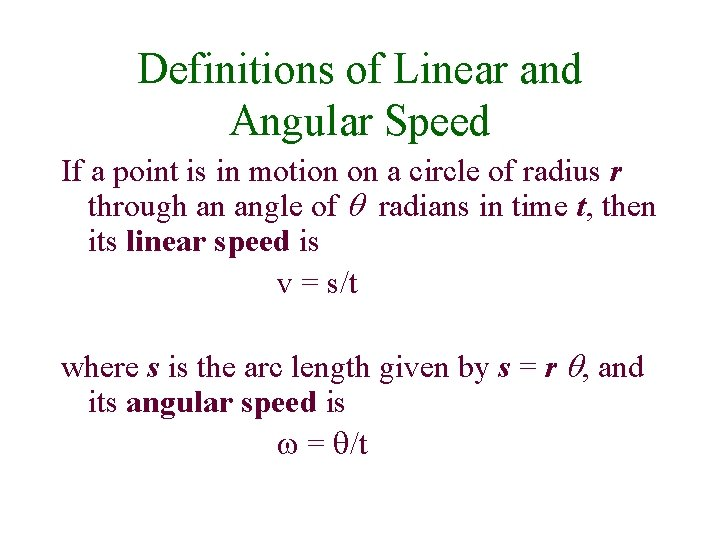Definitions of Linear and Angular Speed If a point is in motion on a circle of radius r through an angle of radians in time t, then its linear speed is v = s/t where s is the arc length given by s = r , and its angular speed is = /t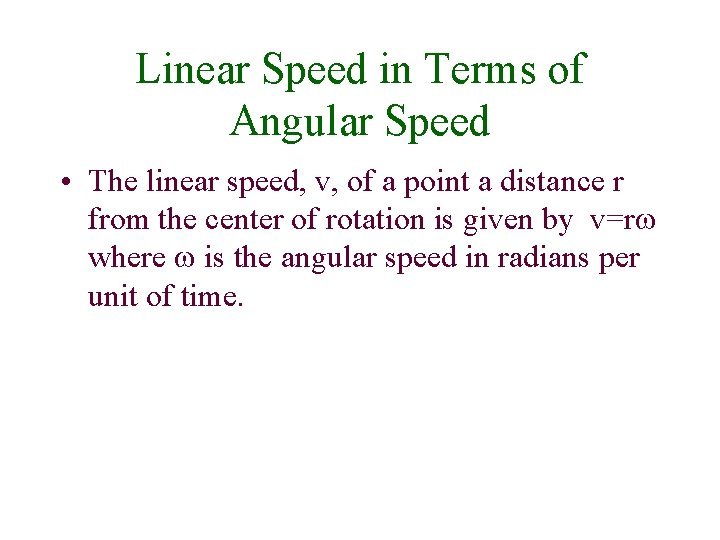Linear Speed in Terms of Angular Speed • The linear speed, v, of a point a distance r from the center of rotation is given by v=r where is the angular speed in radians per unit of time.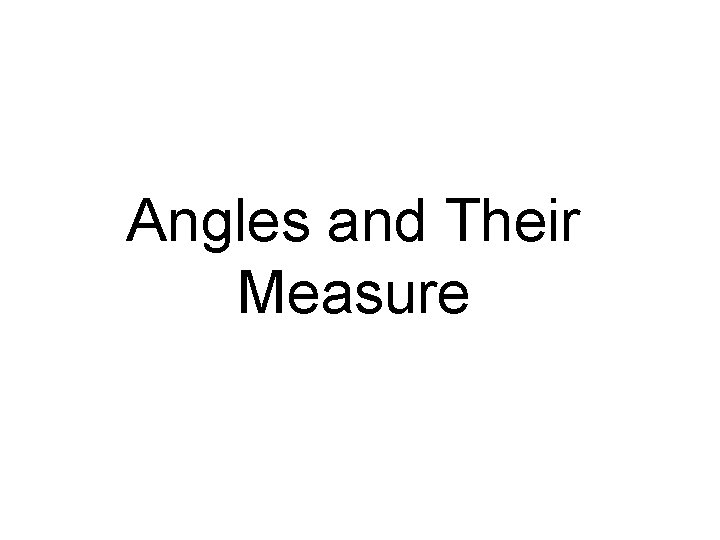Angles and Their Measure